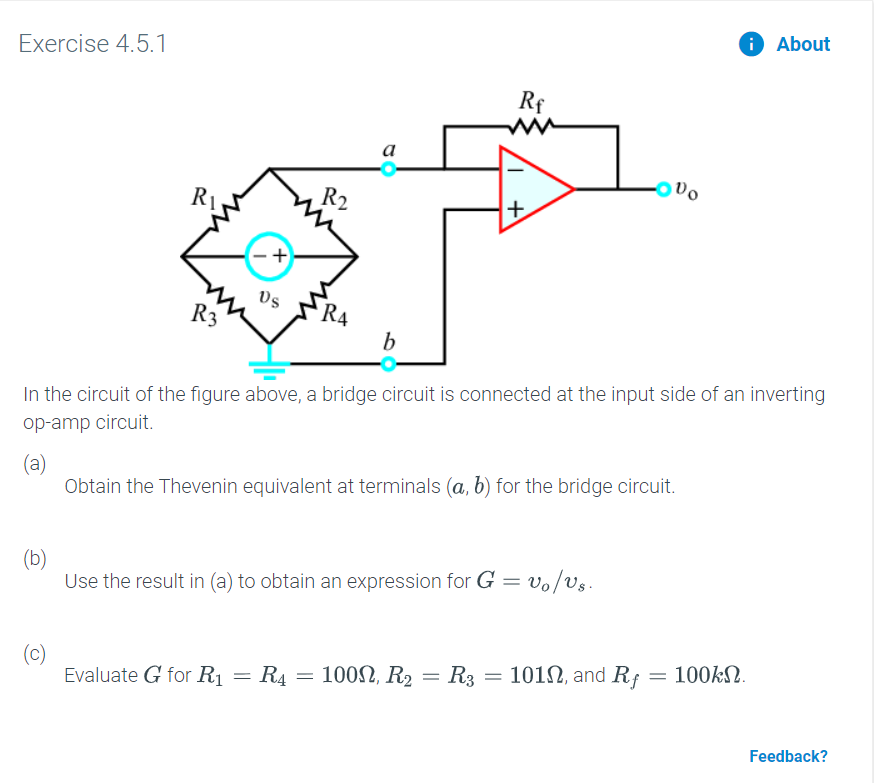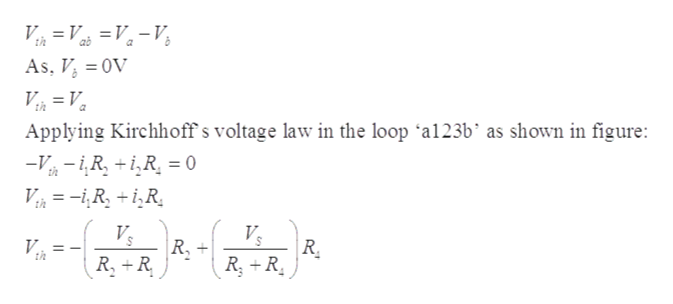# AboutExercise 4.5.1RfwwаR2R4bIn the circuit of the figure above, a bridge circuit is connected at the input side of an invertingop-amp circuit.(a)Obtain the Thevenin equivalent at terminals (a, b) for the bridge circuit.(b)Use the result in (a) to obtain an expression for G = vo/Us(c)Evaluate G for R1101, and Rf = 100kN100, R2 R3R4Feedback?

Question
55 viewshelp_outlineImage TranscriptioncloseAbout Exercise 4.5.1 Rf ww а R2 R4 b In the circuit of the figure above, a bridge circuit is connected at the input side of an inverting op-amp circuit. (a) Obtain the Thevenin equivalent at terminals (a, b) for the bridge circuit. (b) Use the result in (a) to obtain an expression for G = vo/Us (c) Evaluate G for R1 101, and Rf = 100kN 100, R2 R3 R4 Feedback? fullscreen
check_circle

Step 1

(a)

Consider that the current flowing through the upper loop is i1 and the current through the lower loop is i2. Voltage between terminals a and b is the Thevenin voltage Vth. The circuit to find the Thevenin equivalent between the given terminals can be drawn as below:

Step 2

The terminal b is grounded, therefore, Vb = 0V.

The current through the open circuit is zero, thus, the values of current i1 and i2 will be:

Step 3

From the circuit, the Theve...help_outlineImage TranscriptioncloseV = V =V =V th ab As, V OV Applying Kirchhoff s voltage law in the loop 'a123b' as shown in figure: --R,R 0 Vs VE |R, + |R. R R R R fullscreen

### Want to see the full answer?

See Solution

#### Want to see this answer and more?

Solutions are written by subject experts who are available 24/7. Questions are typically answered within 1 hour.*

See Solution
*Response times may vary by subject and question.
Tagged in

### Electrical Engineering